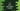# C# program to print a right-angled triangle using any character or number## C# program to print a right-angled triangle using any character or number:

This post will show you how to print a right-angled triangle in C#. The program will use number or any character to print the triangle pattern. It will take the height of the triangle as an input from the user and print the triangle equal to that height.

### Algorithm:

A right-angled triangle of height 5, if we print using * will look as like below:

``````*
* *
* * *
* * * *
* * * * *``````

If you look closely, here,

• The first row has one *.
• The second row has two *, the third row has three * etc.

So, the program will have to print n characters for the nth row. We will use the below algorithm in the program:

• Get the height of the triangle as an input from the user. Store it in a variable.
• Run one loop for height number of times. On each iteration of the loop, we need to run another inner loop to print the characters.
• Run one inner loop for current row number of times. If the outer loop is running for fourth row, the inner loop will run for four times.
• Print the characters in the inner loop.
• Print a new line at the end of each iteration of the outer loop.

### Example 1: C# program to print a right-angled triangle using any character:

The below program uses the above algorithm to print a right-angled triangle using *:

``````namespace Program
{
class Program
{
static void Main(string[] args)
{
int Height;
Console.WriteLine("Enter the height of the triangle: ");

Console.WriteLine();

for (int i = 1; i <= Height; i++)
{
for (int j = 1; j <= i; j++)
{
Console.Write("* ");
}
Console.WriteLine();
}

}
}
}``````

Here,

• It reads the height of the triangle as an input from the user and stores it in the Height variable.
• The outer for loop runs from i = 1 to i = Height, i.e. Height number of times.
• The inner for loop runs for i number of times i.e. for the first line or i = 1, it will run one time, for the second line or i = 2, it will run two times etc.
• The character is printed in the inner for loop.
• At the end of each iteration of the outer for loop, one new line is printed.

If you run this program, it will print output as like below:

``````Enter the height of the triangle:
6

*
* *
* * *
* * * *
* * * * *
* * * * * * ``````

You can also use any other character to print the triangle.

### Example 2: C# program to print a right-angled triangle using numbers:

We can also use numbers to print the triangle. We can use numbers continuously increasing in the whole triangle or increasing in each line, i.e. it will start from 1 on each line.

#### Continuously increasing numbers:

The below program uses continuously increasing numbers to print the triangle:

``````namespace Program
{
class Program
{
static void Main(string[] args)
{
int Height;
Console.WriteLine("Enter the height of the triangle: ");

Console.WriteLine();

int Value = 1;
for (int i = 1; i <= Height; i++)
{
for (int j = 1; j <= i; j++)
{
Console.Write(Value + " ");
Value++;
}
Console.WriteLine();
}

}
}
}``````

It will print output as like below:

``````Enter the height of the triangle:
6

1
2 3
4 5 6
7 8 9 10
11 12 13 14 15
16 17 18 19 20 21 ``````

Here, we are using Value integer variable to hold the current value to print and it is incremented by 1 at the end of each iteration of the loop.

#### Increasing numbers from 1 on each line:

A slight change to the above program requires to print the values from 1 on each row of the triangle.

``````namespace Program
{
class Program
{
static void Main(string[] args)
{
int Height;
Console.WriteLine("Enter the height of the triangle: ");

Console.WriteLine();

int Value;
for (int i = 1; i <= Height; i++)
{
Value = 1;
for (int j = 1; j <= i; j++)
{
Console.Write(Value + " ");
Value++;
}
Console.WriteLine();
}

}
}
}``````

Instead of initializing the Value to 1 before the loops starts, we are re-initializing it to 1 before the inner loop starts. So, the values of each row is printed from 1.

If you run this program, it will print output as like below:

``````Enter the height of the triangle:
6

1
1 2
1 2 3
1 2 3 4
1 2 3 4 5
1 2 3 4 5 6 ``````

### Method 3: Print a right-angled triangle by using a while loop in C#:

We can re-write the above program using while loops. The only difference will be that the loop will check one condition and run its body before it increments the variable used.

``````namespace Program
{
class Program
{
static void Main(string[] args)
{
int Height;
Console.WriteLine("Enter the height of the triangle: ");

Console.WriteLine();

int Value, i = 1, j;
while (i <= Height)
{
Value = 1;
j = 1;
while (j <= i)
{
Console.Write(Value + " ");
Value++;
j++;
}
Console.WriteLine();
i++;
}

}
}
}``````

Here, the values of i and j are initialized before the loops starts. These values are incremented at the end of each loop.

It will give similar results.